# roifill

(Not recommended) Fill in specified region of interest (ROI) polygon in grayscale image

`roifill` is not recommended. Use `regionfill` instead. If you want to define the polygon interactively with `regionfill`, then use `regionfill` either with `roipoly` or with `drawpolygon` followed by `createMask`.

## Syntax

``J = roifill``
``J = roifill(I)``
``J = roifill(I,mask)``
``J = roifill(I,xi,yi)``
``J = roifill(x,y,I,xi,yi)``
``[J,BW] = roifill(___)``
``[x2,y2,J,BW,xi2,yi2] = roifill(___)``
``roifill(___)``

## Description

Use the `roifill` function to fill in a specified region of interest (ROI) polygon in a grayscale image. `roifill` smoothly interpolates inward from the pixel values on the boundary of the polygon by solving Laplace's equation. The boundary pixels are not modified. `roifill` can be used, for example, to erase objects in an image.

````J = roifill` creates an interactive polygon selection tool associated with the image displayed in the current figure. With this syntax and the other interactive syntaxes, the polygon selection tool blocks the MATLAB® command line until you complete the operation. `roifill` fills the selected polygon and returns the filled image, `J`.For more information about using the polygon selection tool to define and fill ROIs, see Interactive Behavior.```
````J = roifill(I)` displays the image `I` in a figure window and creates an interactive polygon tool associated with the image.```
````J = roifill(I,mask)` fills regions in `I` corresponding to the nonzero pixels in the mask. If there are multiple regions, then `roifill` performs the interpolation on each region independently.```

example

````J = roifill(I,xi,yi)` fills in the polygon with vertices defined by X-Y coordinates `xi` and `yi` in the default spatial coordinate system.```
````J = roifill(x,y,I,xi,yi)` defines a nondefault spatial coordinate system using the vectors `x` and `y`. The polygon vertices have coordinates `xi` and `yi` in this coordinate system.```
````[J,BW] = roifill(___)` returns the binary image `BW` with `1`s for pixels corresponding to the interpolated region of `I` and `0`s elsewhere.```
````[x2,y2,J,BW,xi2,yi2] = roifill(___)` also returns the image `XData` and `YData` in `x2` and `y2` and the polygon coordinates in `xi2` and `yi2`.```
````roifill(___)` without an output argument displays the filled image in a new figure window.```

## Examples

collapse all

Read and display a grayscale image.

```I = imread('eight.tif'); imshow(I)```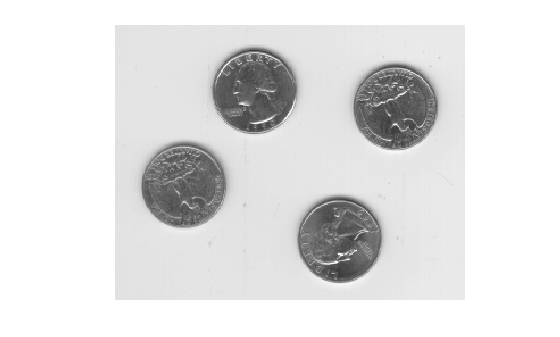Specify the x- and y-coordinates of a polygon that completely surrounds one of the coins in the image.

```x = [222 272 300 270 221 194]; y = [21 21 75 121 121 75];```

Fill the polygon by using the `regionfill` function.

`J = regionfill(I,x,y);`

Display the filled image.

```imshow(J) title('Filled Image with One Fewer Coin')```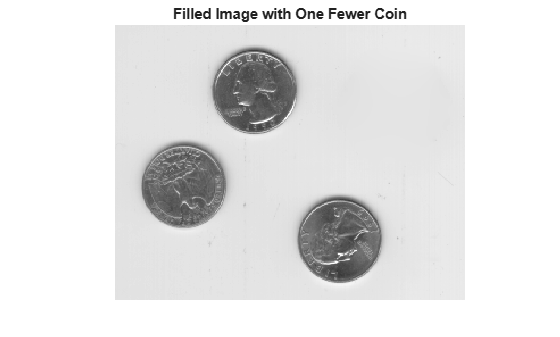## Input Arguments

collapse all

Grayscale image, specified as a numeric matrix.

Data Types: `single` | `double` | `int16` | `uint8` | `uint16`

Mask, specified as a numeric or logical matrix of the same size as the input image, `I`.

Data Types: `single` | `double` | `int8` | `int16` | `int32` | `int64` | `uint8` | `uint16` | `uint32` | `uint64` | `logical`

x- or y-coordinate of polygon vertices, specified as numeric vectors of equal length. If you specify a nondefault coordinate system using the `x` and `y` arguments, then `xi` and `yi` specify coordinates in this coordinate system. Otherwise, `xi` and `yi` specify coordinates in the default coordinate system.

Image extent in world X-Y coordinates, specified as a 2-element numeric vector of the form `[min max]`. The two elements of `x` give the x-coordinates (horizontal) of the first and last columns of image `I`, respectively. The two elements of `y` give the y-coordinates (vertical) of the first and last rows of `I`.

## Output Arguments

collapse all

Filled image, returned as a numeric matrix.

Data Types: `single` | `double` | `int16` | `uint8` | `uint16`

Binary image, returned as a logical matrix of the same size as the input image, `I`.

Data Types: `logical`

x- or y-coordinate of polygon vertices, specified as numeric vectors. `xi` and `yi` are empty if you specify the polygon ROI using the `mask` argument.

Image extent in world X-Y coordinates,, returned as 2-element numeric vectors of the form `[min max]`. If you specify image limits `x` and `y`, then `x2` and `y2` are equal to these values. Otherwise, `x2` and `y2` are equal to the original image `XData` and `YData`.

collapse all

### Interactive Behavior

When you call `roifill` with an interactive syntax, a polygon selection tool opens that enables you to select and adjust polygon vertices interactively using the mouse.

When the polygon tool is active, the pointer changes to cross hairs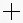when you move the pointer over the image in the figure. Using the mouse, specify the region by selecting vertices of the polygon. You can move or resize the polygon using the mouse. When you are finished positioning and sizing the polygon, fill the polygon by double-clicking, or by right-clicking inside the region and selecting Fill Area from the context menu.

The figure illustrates a polygon defined by multiple vertices.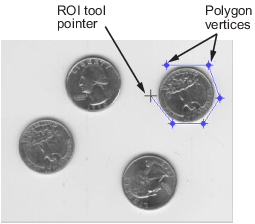Interactive BehaviorDescription
Closing the polygon. (Completing the region-of-interest.)

Use any of the following mechanisms:

• Move the pointer over the initial vertex of the polygon that you selected. The shape changes to a circle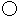. Click either mouse button.

• Double-click the left mouse button. This action creates a vertex at the point under the mouse and draws a straight line connecting this vertex with the initial vertex.

• Click the right mouse button. This action draws a line connecting the last vertex selected with the initial vertex; it does not create a new vertex.

Deleting the polygon

Press Backspace, Escape or Delete, or right-click inside the region and select Cancel from the context menu.

Note: If you delete the ROI, the function returns empty values.

Moving the polygonMove the pointer inside the region. The pointer changes to a fleur. Click and drag the mouse to move the polygon.
Changing the color of the polygonMove the pointer inside the region. Right-click and select Set color from the context menu.
Adding a new vertex.Move the pointer over an edge of the polygon and press the A key. The shape of the pointer changes. Click the left mouse button to create a new vertex at that position on the line.
Moving a vertex. (Reshaping the region-of-interest.)Move the pointer over a vertex. The pointer changes to a circle. Click and drag the vertex to its new position.
Deleting a vertex. Move the pointer over a vertex. The pointer changes to a circle. Right-click and select Delete Vertex from the context menu. This action deletes the vertex and adjusts the shape of the polygon, drawing a new straight line between the two vertices that were neighbors of the deleted vertex.
Retrieving the coordinates of the vertices Move the pointer inside the region. Right-click and select Copy position from the context menu to copy the current position to the Clipboard. Position is an n-by-2 array containing the x- and y-coordinates of each vertex, where n is the number of vertices you selected.

## Version History

Introduced before R2006a